# Right angled

From the right triangle with legs 12 cm and 20 cm we built a square with the same content as the triangle.

How long will be side of the square?

Result

x =  10.95 cm

#### Solution:Leave us a comment of example and its solution (i.e. if it is still somewhat unclear...):

Showing 0 comments:Be the first to comment!#### To solve this example are needed these knowledge from mathematics:

See also our right triangle calculator. See also our trigonometric triangle calculator.

## Next similar examples:

1. Trapezoid - RRFind the area of the right angled trapezoid ABCD with the right angle at the A vertex; a = 3 dm b = 5 dm c = 6 dm d = 4 dm
2. Octagonal mat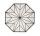Octagonal mat formed from a square plate with a side of 40 cm so that every corner cut the isosceles triangle with leg 3.6 cm. What is the content area of one mat?
3. Chord 2Point A has distance 13 cm from the center of the circle with radius r = 5 cm. Calculate the length of the chord connecting the points T1 and T2 of contact of tangents led from point A to the circle.
4. Ladder 2Ladder 6.4 meters long is positioned in the well such that its lower end is distanced from the wall of the well 1.2 m. The upper part of the ladder is supported on the upper edge of the well. How high is the well?
5. Oil rigOil drilling rig is 23 meters height and fix the ropes which ends are 7 meters away from the foot of the tower. How long are these ropes?
6. Triangle P2Can triangle have two right angles?
7. Triangle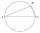Calculate the area of right triangle ΔABC, if one leg is long 14 and its opposite angle is 59°.
8. BaseCompute base of an isosceles triangle, with the arm a=20 cm and a height above the base h=10 cm.
9. Circle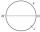On the circle k with diameter |MN| = 61 J lies point J. Line |MJ|=22. Calculate the length of a segment JN.
10. Height 2Calculate the height of the equilateral triangle with side 38.
11. Euklid4Legs of a right triangle have dimensions 244 m and 246 m. Calculate the length of the hypotenuse and the height of this right triangle.
12. Area of RT 2Calculate the area of right triangle whose legs have a length 5.8 cm and 5.8 cm.
13. Square2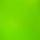Side of the square is a = 6.2 cm, how long is its diagonal?
14. Areaf of ST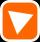It is given square DBLK with side |BL|=13. Calculate area of triangle DKU if vertex U lie on line LB.
15. Center traverseIt is true that the middle traverse bisects the triangle?
16. CalculationHow much is sum of square root of six and the square root of 225?
17. Rectangle vs square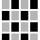The rectangle has dimensions of 13 × 10, square 8 × 8. Which shape has more area and how much above?